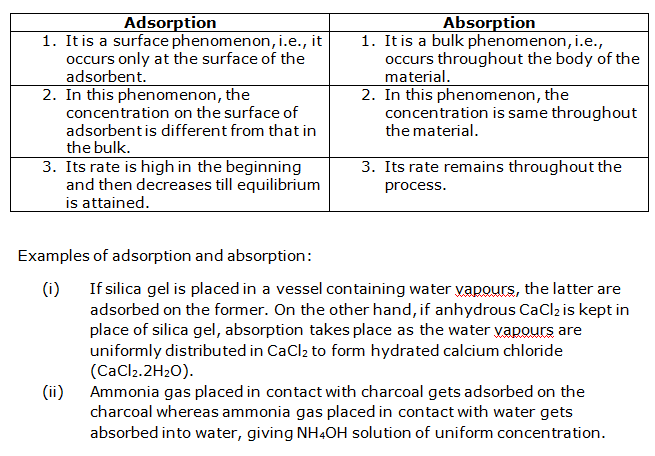# NCERT Solutions for Class 12-science Chemistry Chapter 5 - Surface Chemistry

Page / Exercise

## Chapter 5 - Surface Chemistry Exercise 129

Solution 1Solution 2Solution 3## Chapter 5 - Surface Chemistry Exercise 135

Solution 4Solution 5Solution 6## Chapter 5 - Surface Chemistry Exercise 147

Solution 7Solution 8## Chapter 5 - Surface Chemistry Exercise 148

Solution 1Solution 2Solution 3Solution 4Solution 5Solution 6Solution 7Solution 8Solution 9Solution 10Solution 11Solution 12Solution 13Solution 14Solution 15Solution 16Solution 17Solution 18Solution 19Solution 20Solution 21Solution 22Solution 23Solution 24Solution 25Solution 26Solution 27